# 深入浅出 Java 泛型，一文搞定tips: 建议利用好这个目录，整体把握，局部深入。

@[toc]

## 为什么要有泛型？

• 在编译时进行更强的类型检查

• java 编译器对泛型代码应用强类型检查，如果代码违反了类型安全，则发出错误。修复编译时错误比修复运行时错误更容易，后者可能很难找到。

• 消除显示的类型转换

• 下面没有泛型的代码片段需要强制转换（类型转换是不是很烦人？）:

  List list = new ArrayList();  list.add("hello");  String s = (String) list.get(0);

  List<String> list = new ArrayList<String>();  list.add("hello");  String s = list.get(0);   // no cast

• 使程序员能够实现泛型算法

• 通过使用泛型，程序员可以实现适用于不同类型集合的泛型算法，可以进行定制，并且类型安全且易于阅读。

## 泛型类型简介

### 普通的 Box 类

public class Box {    private Object object;    public void set(Object object) { this.object = object; }    public Object get() { return object; }}

### 泛型版的 Box 类

A generic class is defined with the following format:

class name<T1, T2, ..., Tn> { /* ... */ }

/** * Generic version of the Box class. * @param <T> the type of the value being boxed */public class Box<T> {    // T stands for "Type"    private T t;    public void set(T t) { this.t = t; }    public T get() { return t; }}

### 类型参数命名约定

• E - Element 元素(java 集合框架广泛使用)

• K - Key

• N - Number 数字类型

• T - Type

• V - Value

• S,U,V etc. - 第二个，第三个，第四个类等

### 调用和实例化泛型类型

Box<Integer> integerBox;

类型参数和类型变量术语: 许多开发人员可以混淆地使用术语“类型参数”和“类型变量”，但这两个术语并不相同。编码时，提供类型参数以创建参数化类型。所以 T 在Foo<T> 食物 < t > 是一个类型参数，而 String 字符串在Foo<String> f 是一个类型参数。本课在使用这些术语时遵循这个定义。

Box<Integer> integerBox = new Box<Integer>();

### 菱形写法

Box<Integer> integerBox = new Box<>();

### 多类型参数

public interface Pair<K, V> {    public K getKey();    public V getValue();}public class OrderedPair<K, V> implements Pair<K, V> {    private K key;    private V value;    public OrderedPair(K key, V value) {  this.key = key;  this.value = value;    }    public K getKey()  { return key; }    public V getValue() { return value; }}

Pair<String, Integer> p1 = new OrderedPair<String, Integer>("Even", 8);Pair<String, String>  p2 = new OrderedPair<String, String>("hello", "world");

### 参数化类型

OrderedPair<String, Box<Integer>> p = new OrderedPair<>("primes", new Box<Integer>(...));

## 泛型方法

public class Util {    public static <K, V> boolean compare(Pair<K, V> p1, Pair<K, V> p2) {        return p1.getKey().equals(p2.getKey()) &&               p1.getValue().equals(p2.getValue());    }}public class Pair<K, V> {    private K key;    private V value;    public Pair(K key, V value) {        this.key = key;        this.value = value;    }    public void setKey(K key) { this.key = key; }    public void setValue(V value) { this.value = value; }    public K getKey()   { return key; }    public V getValue() { return value; }}

Pair<Integer, String> p1 = new Pair<>(1, "apple");Pair<Integer, String> p2 = new Pair<>(2, "pear");boolean same = Util.<Integer, String>compare(p1, p2);

## 有界类型参数

public class Box<T> {    private T t;              public void set(T t) {        this.t = t;    }    public T get() {        return t;    }    public <U extends Number> void inspect(U u){        System.out.println("T: " + t.getClass().getName());        System.out.println("U: " + u.getClass().getName());    }    public static void main(String[] args) {        Box<Integer> integerBox = new Box<Integer>();        integerBox.set(new Integer(10));        integerBox.inspect("some text"); // error: this is still String!    }}

Box.java:21: <U>inspect(U) in Box<java.lang.Integer> cannot  be applied to (java.lang.String)                        integerBox.inspect("10");                                  ^1 error

public class NaturalNumber<T extends Integer> {  private T n;  public NaturalNumber(T n)  { this.n = n; }  public boolean isEven() {    return **n.intValue()** % 2 == 0;  }  // ...}

isEven 方法调用 Integer 类中定义的 intValue 方法。

### 有界类型参数在泛型方法中的应用

public static <T> int countGreaterThan(T[] anArray, T elem) {    int count = 0;    for (T e : anArray)        if (e > elem)  // compiler error            ++count;    return count;}

public interface Comparable<T> {    public int compareTo(T o);}

public static <T extends Comparable<T>> int countGreaterThan(T[] anArray, T elem) {    int count = 0;    for (T e : anArray)        if (e.compareTo(elem) > 0)            ++count;    return count;}

## 泛型与继承和子类型概念（可能颠覆你的认知哦）

Object someObject = new Object();Integer someInteger = new Integer(10);someObject = someInteger;   // OK

public void someMethod(Number n) { /* ... */ }someMethod(new Integer(10));   // OKsomeMethod(new Double(10.1));   // OK

Box<Number> box = new Box<Number>();box.add(new Integer(10));   // OKbox.add(new Double(10.1));  // OK

public void boxTest(Box<Number> n) { /* ... */ }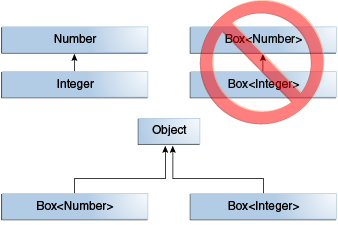Box<Integer> 不是Box<Number>的一个子类型 ，即使 IntegerNumber的子类型。

##### 泛型类和子类型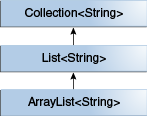interface PayloadList<E,P> extends List<E> {  void setPayload(int index, P val);  ...}

• PayloadList<String,String> PayloadList < String，String >

• PayloadList<String,Integer> PayloadList < String，Integer >

• PayloadList<String,Exception> PayloadList < String，Exception >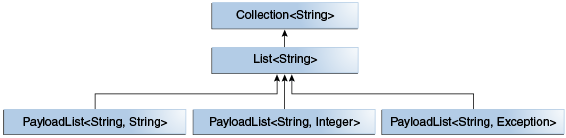## 通配符（Wildcards）

### 无界通配符

• 如果你正在编写一个可以使用 Object 类中提供的功能来实现的方法。

• 当代码在泛型类中使用不依赖于类型参数的方法时。例如，List.size 或 List.clear。事实上，很多使用 Class<T>的方法不依赖于 T，所以 Class<?> 经常被使用（Class<?> is so often used because most of the methods in Class<T> do not depend on T.）；

public static void printList(List<Object> list) {    for (Object elem : list)        System.out.println(elem + " ");    System.out.println();}

printList 的目标是打印任何类型的列表，但是它不能实现这个目标ー它只打印一个 Object 实例列表; 它不能打印 List < integer > 、 List < string > 、 List < double > 等，因为它们不是 List < Object > 的子类型。要编写通用的 printList 方法，请使用 List < ? > :

public static void printList(List<?> list) {    for (Object elem: list)        System.out.print(elem + " ");    System.out.println();}

### 上界通配符

public static void process(List<? extends Foo> list) { /* ... */ }

public static void process(List<? extends Foo> list) {    for (Foo elem : list) {        // ...    }}

public static double sumOfList(List<? extends Number> list) {    double s = 0.0;    for (Number n : list)        s += n.doubleValue();    return s;}

List<Integer> li = Arrays.asList(1, 2, 3);System.out.println("sum = " + sumOfList(li));

Double 值的 List 也可以使用相同的 sumOfList 方法:

List<Double> ld = Arrays.asList(1.2, 2.3, 3.5);System.out.println("sum = " + sumOfList(ld));

### 下界通配符

public static void addNumbers(List<? super Integer> list) {    for (int i = 1; i <= 10; i++) {        list.add(i);    }}

### 通配符和子类继承关系

class A { /* ... */ }class B extends A { /* ... */ }

B b = new B();A a = b;

List<B> lb = new ArrayList<>();List<A> la = lb;   // compile-time error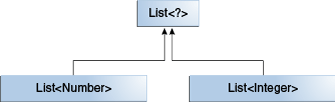List < number > 和 List < integer > 的共同父类是 List < ? > 。学到没？

List<? extends Integer> intList = new ArrayList<>();List<? extends Number>  numList = intList;  // OK. List<? extends Integer> is a subtype of List<? extends Number>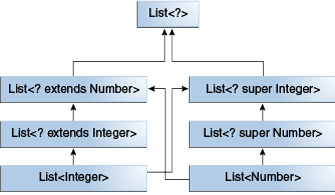### 通配符使用指南

“ in”变量为代码提供数据。想象一个具有两个参数的 copy 方法: copy (src，dest)。Src 参数提供要复制的数据，因此它是“ in”参数。

“ out”变量保存的数据可用于其他地方。在复制示例中，copy (src，dest) ，dest 参数接受数据，因此它是“ out”参数。

• 使用上限通配符定义“ in”变量，使用 extends 关键字。

• 使用下限通配符定义“ out”变量，使用 super 关键字。

• 在可以使用 Object 类中定义的方法访问“ In”变量的情况下，使用无界通配符。

• 如果代码需要同时作为“ In”和“ out”变量访问变量，则不要使用通配符。

// 自然数class NaturalNumber {    private int i;    public NaturalNumber(int i) { this.i = i; }    // ...}// 偶数class EvenNumber extends NaturalNumber {    public EvenNumber(int i) { super(i); }    // ...}

List<EvenNumber> le = new ArrayList<>();List<? extends NaturalNumber> ln = le;ln.add(new NaturalNumber(35));  // compile-time error

• 你可以添加 null

• 你可以执行 clear

• 你可以获取迭代器 iterator 和 remove

• 你可以捕获通配符并编写从列表中读取的元素。

end，其实并没有全部翻译，而是满足目前的需求：一个是系统化，一个是常用知识点。### 猴哥一一 cium

Java 从业者, 目前就职于杭州。 有个公众号（不过还没写东西，哈哈）：猴哥一一

## 评论